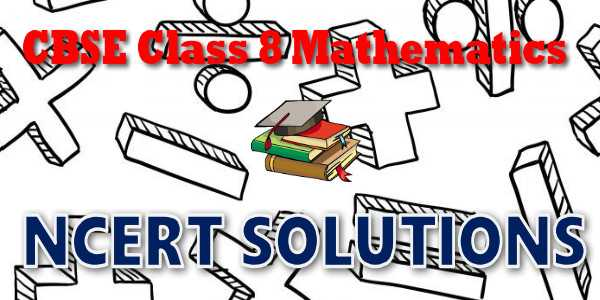No products in the cart.

# CBSE - Class 08 - Mathematics - Cubes and Cube Roots - NCERT Solutions

## NCERT Solutions for Mathematics Cubes and Cube Roots### Student Subscription

##### Unlock the exclusive content designed for the toppers

We will update content for this category shortly. Please visit this category after few days or subscribe to our newsletter by email for latest updates

# NCERT solutions for class 8 Mathematics Cubes and Cube Roots## NCERT Class 8 Mathematics Chapter-wise Solutions

Maths Part I

• Chapter 1 - Rational Numbers
• Chapter 2 - Linear Equations in One Variable
• Chapter 3 - Understanding Quadrilaterals
• Chapter 4 - Practical Geometry
• Chapter 5 - Data Handling
• Chapter 6 - Squares and Square Roots
• Chapter 7 - Cubes and Cube Roots
• Chapter 8 - Comparing Quantities
• Chapter 9 - Algebraic Expressions and Identities
• Chapter 10 - Visualising Solid Shapes
• Chapter 11 - Mensuration
• Chapter 12 - Exponents and Powers
• Chapter 13 - Direct and Inverse Proportions
• Chapter 14 - Factorisation
• Chapter 15 - Introduction to Graphs
• Chapter 16 - Playing with Numbers

## CBSE class 8th Mathematics have two books. Each book has chapters and topics.

• NCERT Mathematics Book Class 8 Part-1

Here is the list of topics covered under each chapter of class 8 Mathematics NCERT text book.

• Exercise 1.1
• Exercise 1.2

• Exercise 2.1
• Exercise 2.2
• Exercise 2.3
• Exercise 2.4
• Exercise 2.5
• Exercise 2.6

• Exercise 3.1
• Exercise 3.2
• Exercise 3.3
• Exercise 3.4

• Exercise 4.1
• Exercise 4.2
• Exercise 4.3
• Exercise 4.4
• Exercise 4.5

• Exercise 5.1
• Exercise 5.2
• Exercise 5.3

• Exercise 6.1
• Exercise 6.2
• Exercise 6.3
• Exercise 6.4

• Exercise 7.1
• Exercise 7.2

• Exercise 8.1
• Exercise 8.2
• Exercise 8.3

• Exercise 9.1
• Exercise 9.2
• Exercise 9.3
• Exercise 9.4
• Exercise 9.5

### 10. Visualising Solid Shapes

• Exercise 10.1
• Exercise 10.2
• Exercise 10.3

### 11. Mensuration

• Exercise 11.1
• Exercise 11.2
• Exercise 11.3
• Exercise 11.4

### 12. Exponents and Powers

• Exercise 12.1
• Exercise 12.2

### 13. Direct and Inverse Proportions

• Exercise 13.1
• Exercise 13.2

### 14. Factorisation

• Exercise 14.1
• Exercise 14.2
• Exercise 14.3
• Exercise 14.4

### 15. Introduction to Graphs

• Exercise 15.1
• Exercise 15.2
• Exercise 15.3

### 16.Playing with Numbers

• Exercise 16.1
• Exercise 16.2

## NCERT Solutions Chapter 7 Cubes and Cube Roots

This is a story about one of India’s great mathematical geniuses, S. Ramanujan. Once
another famous mathematician Prof.G.H. Hardy came to visit him in a taxi whose number was 1729. While talking to Ramanujan, Hardy described this number “a dull number”. Ramanujan quickly pointed out that 1729 was indeed interesting. He said it is the smallest number that can be expressed as a sum of two cubes in two different ways:
1729 = 1728 + 1 = 123 + 13
1729 = 1000 + 729 = 103 + 93

1729 has since been known as the Hardy – Ramanujan Number,
even though this feature of 1729 was known more than 300 years
before Ramanujan.

## NCERT Solutions for Class 8th Mathematics

NCERT Solutions Class 8 Mathematics PDF (Download) Free from myCBSEguide app and myCBSEguide website. Ncert solution class 8 Mathematics includes text book solutions from  part . NCERT Solutions for CBSE Class 8 Mathematics have total 16 chapters. Class 8 Mathematics ncert Solutions in pdf for free Download are given in this website. Ncert Mathematics class 8 solutions PDF and Mathematics ncert class 8 PDF solutions with latest modifications and as per the latest CBSE syllabus are only available in myCBSEguide.

## NCERT Solutions for Class 5

NCERT Solutions for Class 4

## NCERT Solutions for Class 3## myCBSEguide

Trusted by 1 Crore+ Students## Test Generator

Create papers online. It's FREE.## CUET Mock Tests

75,000+ questions to practice only on myCBSEguide app